Select Page

# MCQ Solutions for CBSE 12 Science Maths Relations and Functions in English

MCQ Solutions for CBSE 12 Science Maths Relations and Functions in English to enable students to get Solutions in a narrative video format for the specific question.

Expert Teacher provides MCQ Solutions for CBSE 12 Science Maths Relations and Functions through Video Solutions in English language. This video solution will be useful for students to understand how to write an answer in exam in order to score more marks. This teacher uses a narrative style for a question from Relations and Functions not only to explain the proper method of answering question, but deriving right answer too.

Please find the question below and view the Solution in a narrative video format.

Question:

Solution Video in English:

You can select video Solutions from other languages also. Please check Solutions in ( Hindi )

## Similar Questions from CBSE, 12th Science, Maths, Relations and Functions

Question 1 : Let * be the binary operation on N given by a * b = LCM of a and b. Find the identity of * in N? (View Answer Video)

Question 2 : Letbe defined as f(x) = 3x. Choose the correct answer. (View Answer Video)

Question 3 : Let * be the binary operation on N given by a * b = LCM of a and b. Find 20 * 16. (View Answer Video)

Question 4 : Let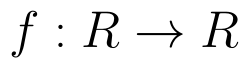be defined as f(x) = 3x.  (View Answer Video)

Question 5 :  A function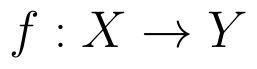is surjective if and only if , (View Answer Video)

### Matrices

Question 1 : Find the transpose of the matrix: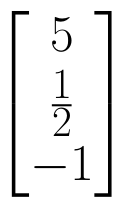. (View Answer Video)

Question 2 : Compute: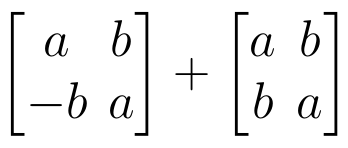. (View Answer Video)

Question 3 : If A is a square matrix such that,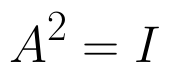then find the simplified value of: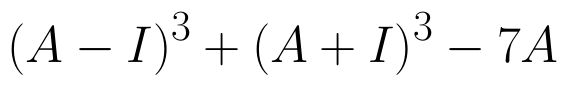. (View Answer Video)

Question 4 : Find the value of y,  from the equation: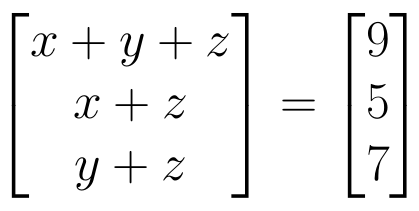. (View Answer Video)

Question 5 :  Find the value of t, if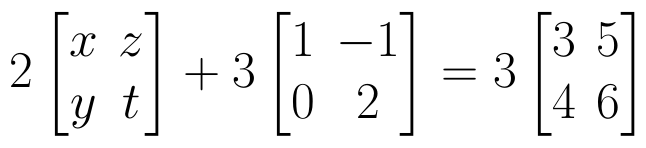(View Answer Video)

### Application of Integrals

Question 1 : Using integration, find the area of the region bounded by the curves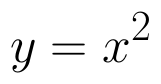and y = x. (View Answer Video)

Question 2 : Using integration, find the area of the triangle ABC, where A is (2,3), B is (4,7) and C is (6,2). (View Answer Video)

Question 3 : Using integration, find the area of the triangle formed by a positive x-axis and tangent and normal to the circle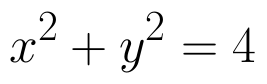at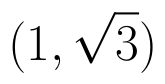. (View Answer Video)

Question 4 : Find the area of the region bounded by the curve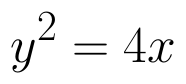and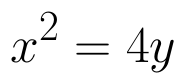. (View Answer Video)

Question 5 : Find the area of the region bounded by the curves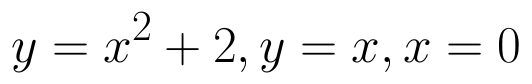and x = 3. (View Answer Video)

### Determinant

Question 1 : If x, y, z are non-zero real numbers, then the inverse of matrix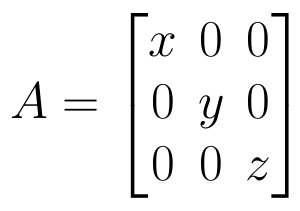is, (View Answer Video)

Question 2 : Let A be a non-singular square matrix of order 3 * 3. Then | adj A | is equal to: (View Answer Video)

Question 3 : Evaluate the determinant: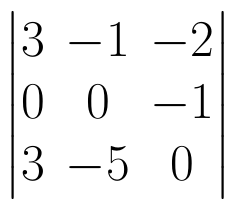. (View Answer Video)

Question 4 : A system of linear equations AX = B is said to be inconsistent, if the system of equations has, (View Answer Video)

Question 5 : Let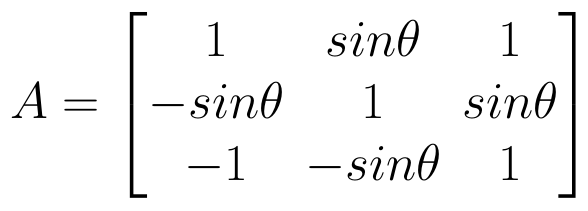, where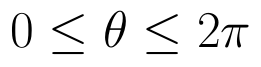. THhen (View Answer Video)